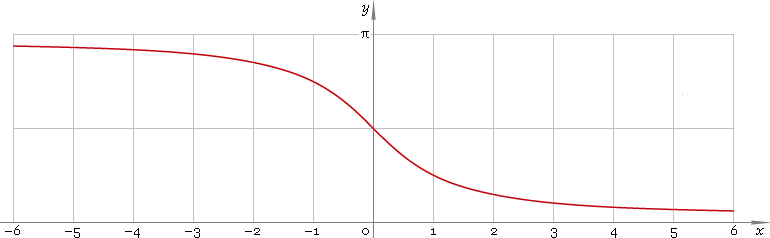The Art of Interface

# arccot or arcctg — trigonometric arc cotangent function

Category. Mathematics.

Abstract. Trigonometric arc cotangent: definition, plot, properties, identities and table of values for some arguments.

## 1. Definition

Arc cotangent is inverse of the cotangent function.

## 2. Plot

Arc cotangent is monotone function defined everywhere on real axis. Its plot is depicted below — fig. 1.Fig. 1. Plot of the arc cotangent function y = arccotx.

Function codomain is limited to the range (0, π).

## 3. Identities

Complementary angle:

arctanx + arccotx = π/2

and as consequence:

arccot tan φ = π/2 − φ

Negative argument:

arccot(−x) = π − arccotx

Reciprocal argument:

arccot(1/x) = arctanx for x > 0,
arccot(1/x) = arctanx + π for x < 0

Sum and difference:

arccotx + arccoty = arccot[(xy − 1) /(x + y)]
arccotx − arccoty = arccot[(xy + 1) /(yx)]

Some argument values:

Argument xValue arccotx
0π/2
2 − √35π/12
√(1 − 2 /√5)2π/5
√2 − 13π/8
√3 /3π/3
√(5 − 2 √5)3π/10
1π/4
√(1 + 2 /√5)π/5
√3π/6
√2 + 1π/8
√(5 + 2 √5)π/10
2 + √3π/12
Table 1. Arc cotangent for some argument values.

## 4. Support

Trigonometric arc cotangent function arccot or arcctg of the real argument is supported by free version of the Librow calculator.

Trigonometric arc cotangent function arccot or arcctg of the complex argument is supported by professional version of the Librow calculator.

## 5. How to use

To calculate arc cotangent of the number:

``arccot(-1);``

To calculate arc cotangent of the current result:

``arccot(rslt);``

To calculate arc cotangent of the number x in memory:

``arccot(mem[x]);``